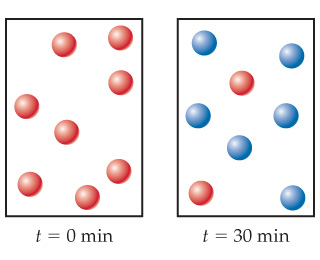# Problem: After four half-life periods for a first-order reaction, what fraction of reactant remains?

###### FREE Expert Solution

We’re being asked to calculate the fraction of the reactant remaining after 4 half-lives

Recall that half-life is the time needed for the amount of a reactant to decrease by 50% or one-half. One way to determine the amount remaining after x half-lives is:

The half-life of a first order reaction is given by:

$\overline{){{\mathbf{t}}}_{\mathbf{1}\mathbf{/}\mathbf{2}}{\mathbf{=}}\frac{\mathbf{ln}\mathbf{2}}{\mathbf{k}}}$

95% (193 ratings)###### Problem DetailsAfter four half-life periods for a first-order reaction, what fraction of reactant remains?